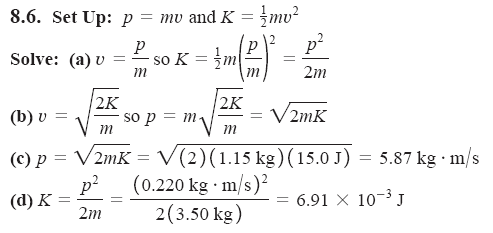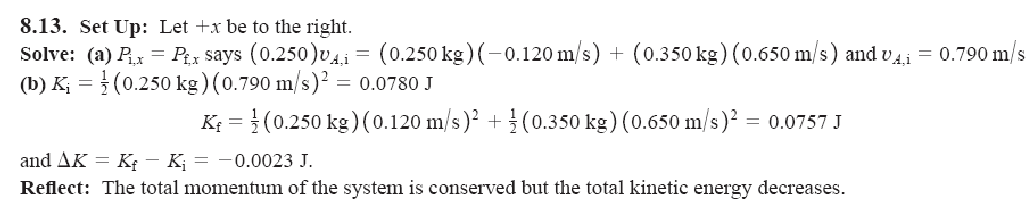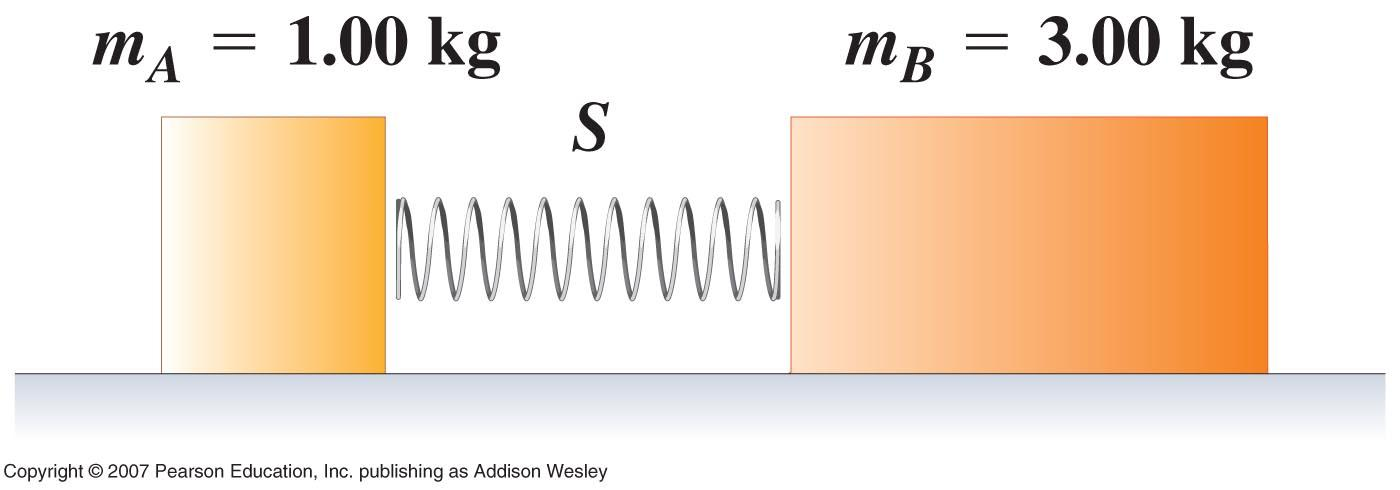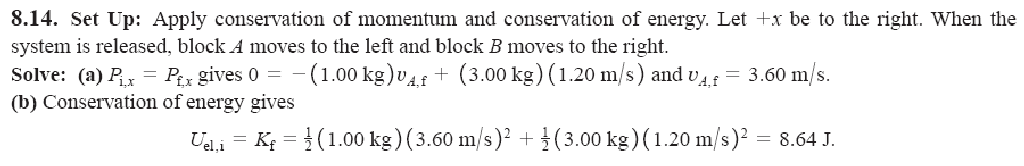# Recitation week 11 conservation of momentum some Useful Relationships

 RECITATION WEEK 11 CONSERVATION OF MOMENTUM Some Useful Relationships. The following relationships between the momentum and kinetic energy of an object can be very useful for calculations: If an object of mass m has momentum of magnitude p and kinetic energy K, show that (a) K=(p2 /2m), and (b) p=sqroot(2mk) (c) Find the momentum of a 1.15 kg ball that has 15.0 J of kinetic energy. (d) Find the kinetic energy of a 3.50 kg cat that has 0.220kg.m/s of momentum.8-13 On a frictionless, horizontal air table, puck A (with mass 0.250kg) is moving toward puck B (with mass 0.350 kg), which is initially at rest. After the collision, puck A has a velocity of 0.120m/s to the left, and puck B has a velocity of 0.650 m/s to the right. (a) What was the speed of puck A before the collision? (b) Calculate the change in the total kinetic energy of the system that occurs during the collision?Block A in the figure has mass 1.00kg, and block B has mass 3.00kg. The blocks are forced together, compressing a spring S between them; then the system is released from rest on a level, frictionless surface. The spring, which has negligible mass, is not fastened to either block and drops to the surface after it has expended. Block B acquires a speed of 1.20 m/s. (a) What is the final speed of block A? (b) How much potential energy was stored in the compressed spring?Share with your friends: